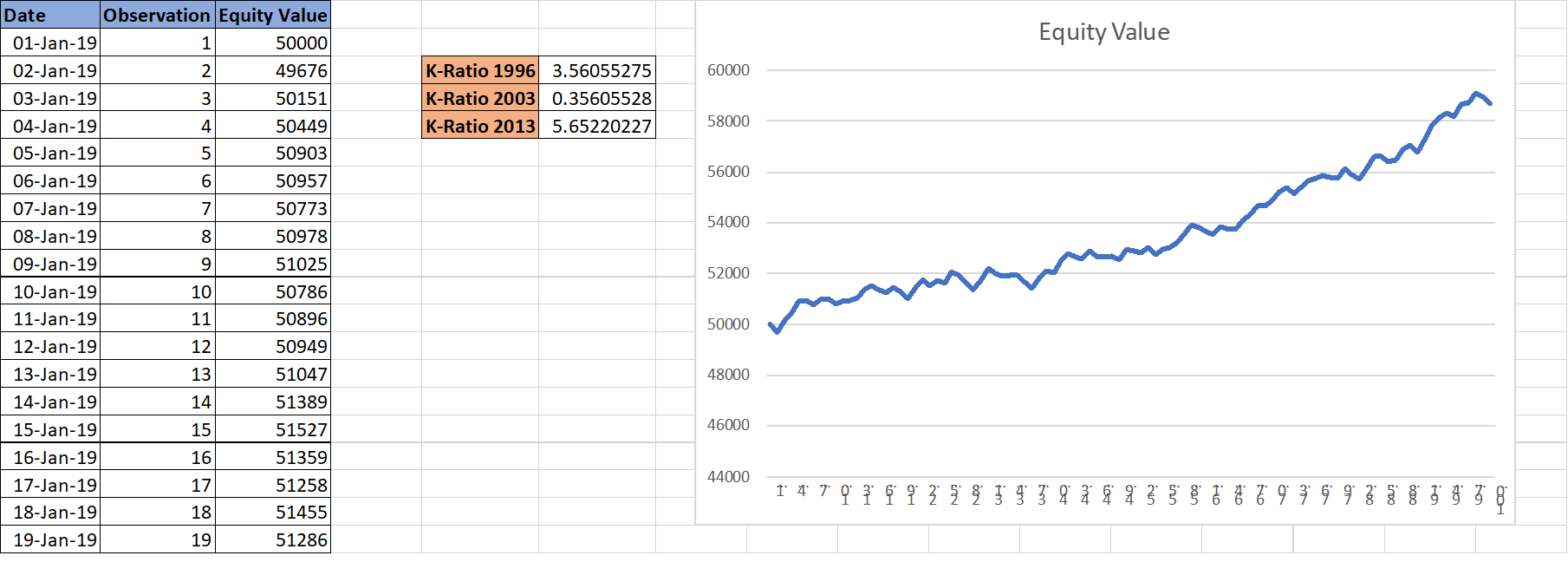# Understanding K-Ratio : Excel Sheet Included

K-Ratio is a statistical measure that determines the consistency of your returns over time. It not only factors in the start and end capital but also considers how the capital grew over a period of time. It is a very important metric to evaluate the performance of any trading system, in fact, you’ll find this in the backtest report of many popular softwares.

K-Ratio was developed by a famous statistician and derivatives trader Lars Kestner in 1996. He published this unconventional idea in an article titled “Measuring System Performance” in stock and commodities magazine. During that period there existed a gap on how to effectively analyze the returns and measure a trading system, and K-ratio effectively bridged this gap.

## K-Ratio Calculation

The numerator of K-ratio estimates the returns, while denominator estimates risks. So, a higher positive value is desirable for the best performing trading system.

In statistical terms, the numerator is the slope of the best-fit regression line over a cumulative return series while denominator represents standard error of the same regression line.

The formula for calculation of K-Ratio was revised 3 times to improve its accuracy. Below are the three versions:## K-Ratio Excel Sheet

We have built an excel sheet that calculates K-Ratio for a given equity curve. The equity curve is derived using the cumulative returns over a period of time.

All the 3 versions of K-Ratio are automatically calculated in this Excel sheet. All you need to input is your equity value for a time-series.

See the screenshot below: